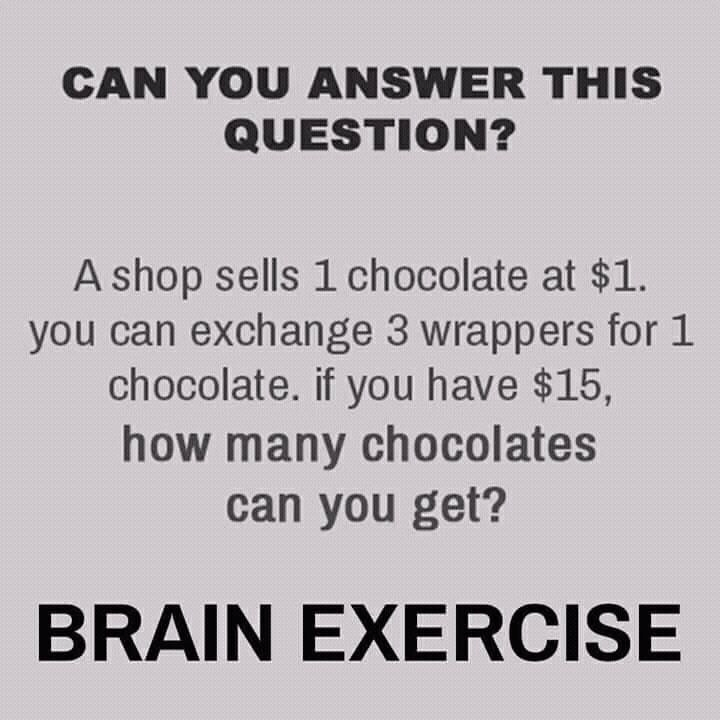Categories

# Puzzle – how many chocolates can you get?

Solving math riddles and puzzles is real fun. It is good time-pass activity with some brain exercise. Lets see if you can solve this math puzzle. It is not that straight forward as it looks, spend some time on it so you can solve it correctly. Match your answer with the one we have published here.A shop sells a chocolate at \$1.

You can exchange 3 wrappers for 1 chocolate.

If you have \$15, how many chocolates can you get?

Also Try: Who’s Telling the Truth?

You can get total 22 chocolates.

Explanation:

1 chocolate for \$1, so

A = 15 chocolates for \$15.

If you return 15 wrappers, so

B = you can get 5 more chocolates (15/3).

Return 3 wrappers out of 5, so

C =  you get 1 more chocolate (3/3)

Now return 2 remaining wrappers + 1 wrapper for chocolate you got in C. So,

D = 1 Chocolate ((2+1)/3)

Total Chocolates = A + B + C + D = 15 + 5 + 1 + 1 =22

Also Try: Puzzle – Find Hidden Face